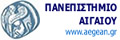Design, Operations, & Production Systems Lab (DeOPSys)Department of Financial & Management EngineeringUniversity of the Aegean# Amanatidis LazarosThe focus of the current master thesis is set on the definition of the frequency of service in a distribution network by maximizing the profit in a specific time window. The problem is modeled using integer mathematical programming and is solved using the Brach and Bound algorithm in order to find the optimal solution.
This problem has already been solved within an Undergraduate thesis developed by the DeOpSys team of the University of the Aegean by Asimakopoulos (2006). In that effort the focus was given in solving practical sized problems using a specific heuristic algorithm. The actual solution of the problem using the Branch and Bound algorithm was narrowed down to a small number of clients due to the high complexity of the problem (number of variables and constraints).
Therefore, primary goal of this thesis is to enhanced solution method in order to have better computational times than the previous approach and be able to solve larger problems.
More specifically, we developed a software tool in C++ that implements the above mentioned algorithm and provides:
• Better solution times for problems being solved by Asimakopoulos (2006) application
• Solution of bigger problems while keeping the same algorithm
Finally, a windows application as interface of the algorithm was implemented. This application provides interface for entering the project’s input data and representation of coordinates in geographical maps (Google maps) as well as generation of the results (route and cost) on  the same maps.

Keywords: Retail distribution network, branch and bound, tsp

People: